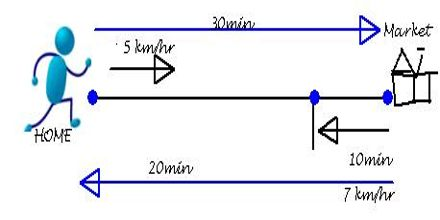Mathematic

# Motion in a Straight Line: Graphically PresentBasic purpose of this lecture is to present on Motion in a Straight Line Graphically. It is a tutorial based lecture. By graphing some examples of motion at a constant velocity. Three different curves are included on the graph to the right, each with an initial displacement of zero. Summary of this lecture are: The gradient of a distance-time graph gives the speed; the gradient of a velocity-time graph gives the acceleration and the area under a velocity-time graph gives the distance travelled.